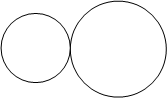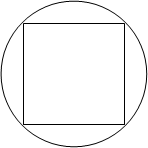### Questions of Test: GRE Quantitative Section practice test

 1 ```Direction: Each of the Questions 1-15 consists of two quantities, one in Column A and one in Column B. You are to compare the two quantities. Column A Column B y2 - z2 = 21 y = 5  z2 2 ``` 2 ```Direction: Each of the Questions 1-15 consists of two quantities, one in Column A and one in Column B. You are to compare the two quantities. Column A Column B a = -2.942 and [a] is the greatest integer that is less than a   [a] -2 ``` 3 ```Direction: Each of the Questions 1-15 consists of two quantities, one in Column A and one in Column B. You are to compare the two quantities. Column A Column B x2 + y2 < 4 x2 - y2 4 ``` 4 ```Direction: Each of the Questions 1-15 consists of two quantities, one in Column A and one in Column B. You are to compare the two quantities. Column A Column B m and n are prime numbers less than 100. m divided by n gives a remainder of 2. n divided by m gives a remainder of 3 m n ``` 5 ```Direction: Each of the Questions 1-15 consists of two quantities, one in Column A and one in Column B. You are to compare the two quantities. Column A Column BThe two circles intersect each other at exactly one point. The shortest distance between the centers of the two circles The sum of the circumference of both circles divided by 2π ``` 6 ```Direction: Each of the Questions 1-15 consists of two quantities, one in Column A and one in Column B. You are to compare the two quantities. Column A Column B |-1| ≥ q q -1 ``` 7 ```Direction: Each of the Questions 1-15 consists of two quantities, one in Column A and one in Column B. You are to compare the two quantities. Column A Column B p is a multiple of 6 The remainder when p is divided by 3 1 ``` 8 ```Direction: Each of the Questions 1-15 consists of two quantities, one in Column A and one in Column B. You are to compare the two quantities. Column A Column BThe square is inscribed inside the circle. The diameter of the circle The side length of the square ``` 9 ```Direction: Each of the Questions 1-15 consists of two quantities, one in Column A and one in Column B. You are to compare the two quantities. Column A Column B The area of a rectangle with a perimeter of 10 The area of a triangle with a perimeter of 10 ``` 10 ```Direction: Each of the Questions 1-15 consists of two quantities, one in Column A and one in Column B. You are to compare the two quantities. Column A Column B The price of m grams of mineral which costs q dollars per gram The price of m/5 grams of jewelry which costs 10q dollars per gram ```